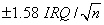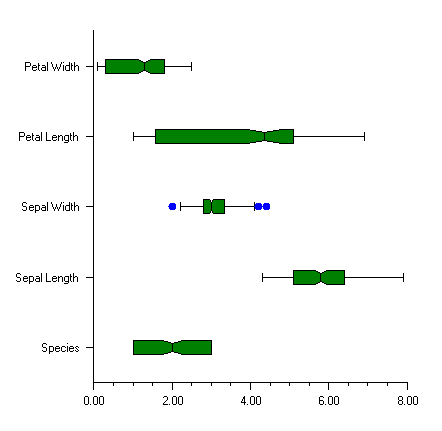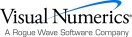## Box Plot

BoxPlot plots are used to show statistics about multiple groups of observations.

For each group of observations, the box limits represent the lower quartile (25-th percentile) and upper quartile (75-th percentile). The median is displayed as a line across the box. Whiskers are drawn from the upper quartile to the upper adjacent value, and from the lower quartile to the lower adjacent value.

Optional notches may be displayed to show a 95 percent confidence interval about the median, atwhere IRQ is the interquartile range and n is the number of observations. Outside and far outside values may be displayed as symbols. Outside values are outside the inner fence. Far out values are outside the outer fence.

The BoxPlot has several child nodes. Any of these nodes can be disabled by setting their IsVisible property to false.

The Bodies node has the main body of the box plot elements. Its fill attributes determine the drawing of (notched) rectangle (see Fill Area Attributes). Its line attributes determine the drawing of the median line. The width of the box is controlled by the MarkerSize attribute (see Attribute MarkerSize).

The Whiskers node draws the lines to the upper and lower quartile. Its drawing is affected by the marker attributes.

The FarMarkers node holds the far markers. Its drawing is affected by the marker attributes.

The OutsideMarkers node holds the outside markers. Its drawing is affected by the marker attributes.

### Example

In this example, the Fisher iris data set is read from a file and a Box plot is created from data. The data is in a file called FisherIris.csv in the same directory as this class.

The y-axis labels are taken from the column names.

The boxes are colored green; the markers are all filled circles. The outside markers are blue and the far outside markers would be red, if there were any.using Imsl.Chart2D;

using System.Drawing;

using System.IO;

public class SampleBoxPlot : FrameChart {

private double[][] irisData;

private string[] labels;

public SampleBoxPlot() {

Chart chart = this.Chart;

AxisXY axis = new AxisXY(chart);

BoxPlot boxPlot = new BoxPlot(axis, irisData);

boxPlot.BoxPlotType = BoxPlot.BOXPLOT_TYPE_HORIZONTAL;

boxPlot.SetLabels(labels);

boxPlot.Bodies.FillColor = Color.Green;

boxPlot.MarkerType = BoxPlot.MARKER_TYPE_FILLED_CIRCLE;

boxPlot.OutsideMarkers.MarkerColor = Color.Blue;

boxPlot.FarMarkers.MarkerColor = Color.Red;

boxPlot.Notch = true;

}

public static void Main(string[] argv) {

System.Windows.Forms.Application.Run(new SampleBoxPlot());

}

int nColumns = 5;

int nObs = 150;

irisData = new double[nColumns][];

for (int i = 0; i < nColumns; i++) irisData[i] =

new double[nObs];

string line;

string[] tokens;

int lineCount = 0;

labels = line.Split(',');

for (int i = 0; i < nObs; i++) {

tokens = line.Split(',');

for (int j = 0; j < nColumns; j++) {

irisData[j][lineCount] =

double.Parse(tokens[j].Trim());

}

lineCount++;

}

}

}Visual Numerics - Developers of IMSL and PV-WAVEhttp://www.vni.com/PHONE: 713.784.3131FAX:713.781.9260